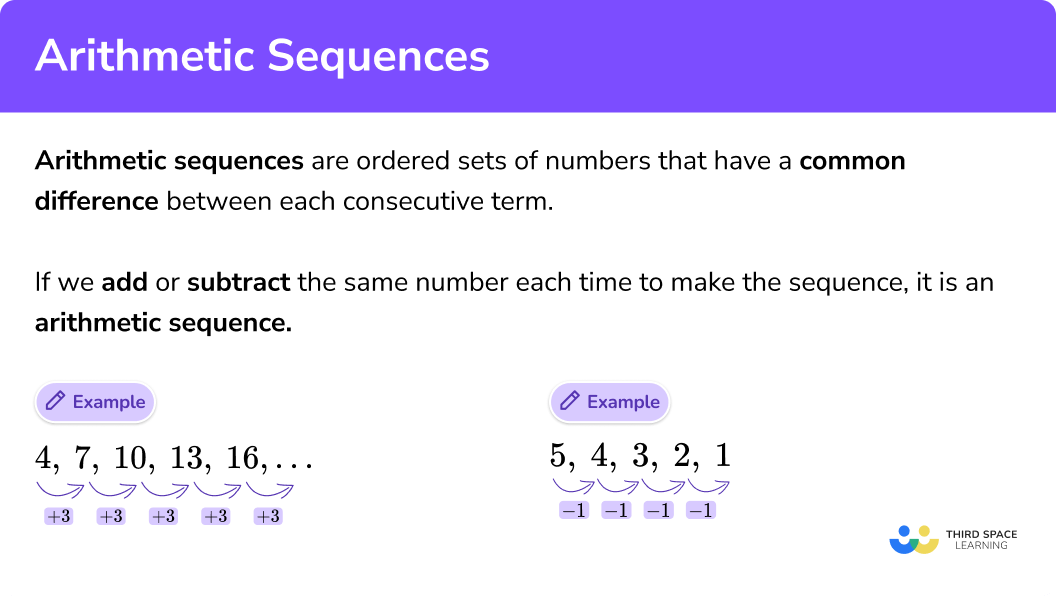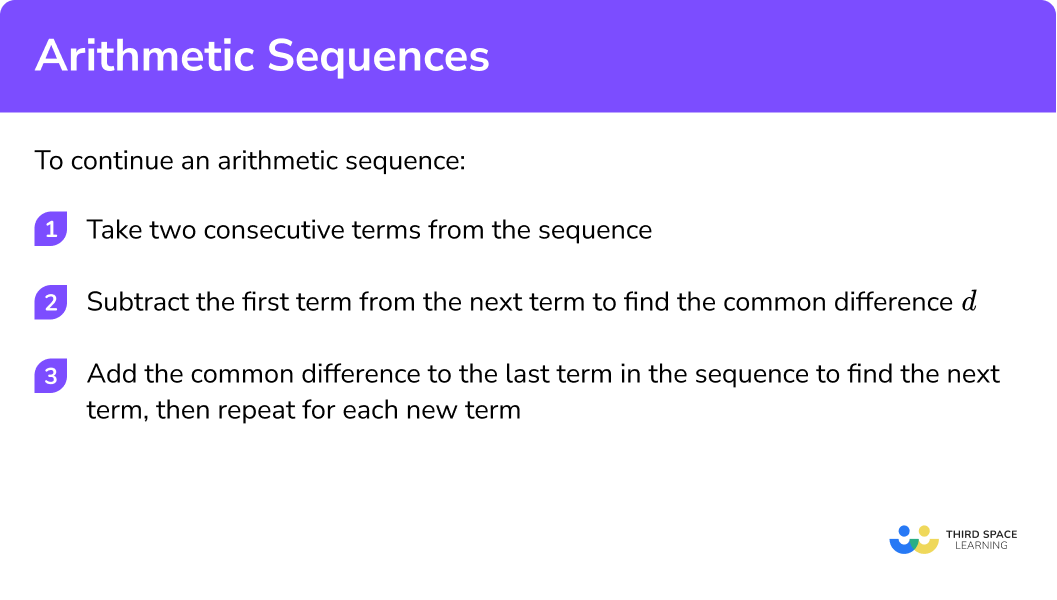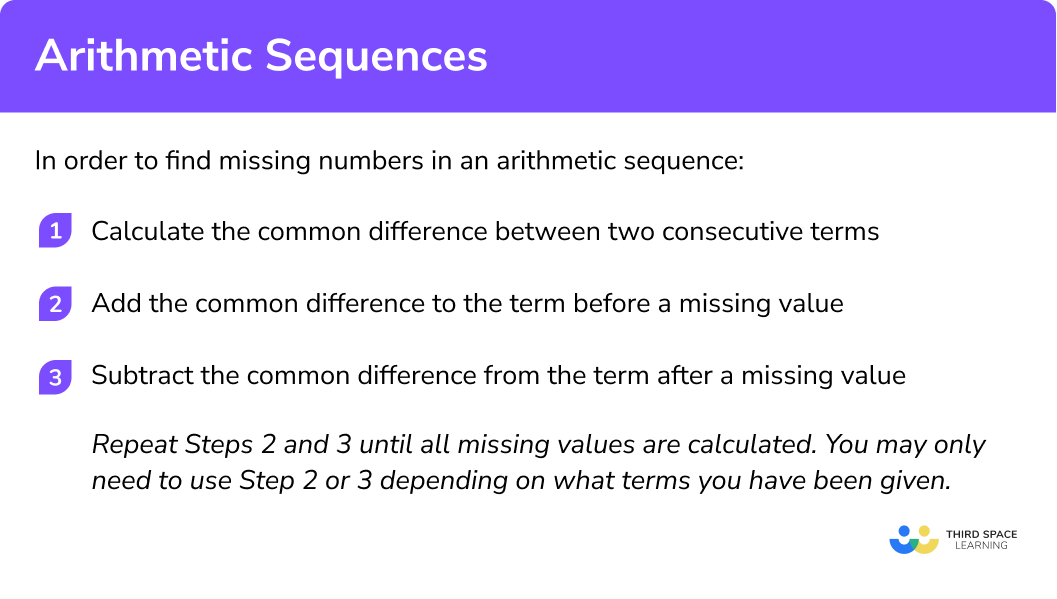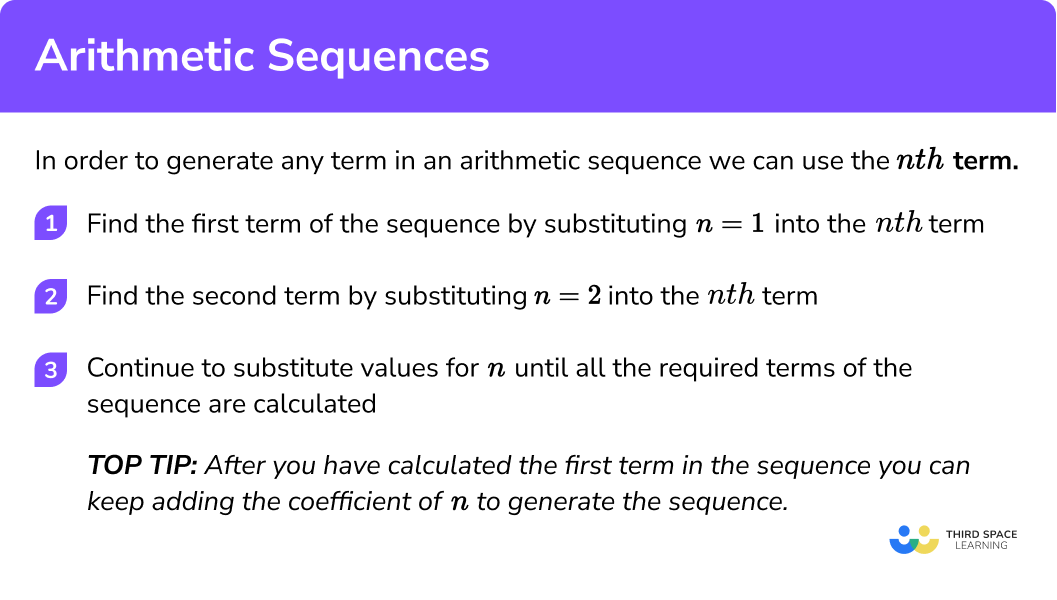GCSE Maths Algebra Sequences

Arithmetic Sequence

# Arithmetic Sequence

Here we will learn what an arithmetic sequence is, how to continue an arithmetic sequence, how to find missing terms in an arithmetic sequence and how to generate an arithmetic sequence.

At the end you’ll find arithmetic sequence worksheets based on Edexcel, AQA and OCR exam questions, along with further guidance on where to go next if you’re still stuck.

## What is an arithmetic sequence?

An arithmetic sequence is an ordered set of numbers that have a common difference between each consecutive term.

For example in the arithmetic sequence 3, 9, 15, 21, 27, the common difference is 6.

An arithmetic sequence can be known as an arithmetic progression. The difference between consecutive terms is an arithmetic sequence is always the same.

If we add or subtract by the same number each time to make the sequence, it is an arithmetic sequence.

The term-to-term rule tells us how we get from one term to the next.

Here are some examples of arithmetic sequences:

## Linear sequences

Arithmetic sequences are also known as linear sequences. If we represented an arithmetic sequence on a graph it would form a straight line as it goes up (or down) by the same amount each time. Linear means straight.

### What are arithmetic sequences?## How to continue an arithmetic sequence

In order to continue an arithmetic series, you should be able to spot, or calculate, the term-to-term rule. This is done by subtracting two consecutive terms to find the common difference.

The common difference for an arithmetic sequence is the same for every consecutive term and can determine whether a sequence is increasing or decreasing.

1. Take two consecutive terms from the sequence.
2. Subtract the first term from the next term to find the common difference d.
3. Add the common difference to the last term in the sequence to find the next term. Repeat for each new term.

### Explain how to continue an arithmetic sequence in 3 steps### Related lessons on sequences

Arithmetic sequence is part of our series of lessons to support revision on sequences. You may find it helpful to start with the main sequences lesson for a summary of what to expect, or use the step by step guides below for further detail on individual topics. Other lessons in this series include:

### Arithmetic sequence formula

The arithmetic sequence formula is:

Where,

a_{n} is the nth term (general term)

a_{1} is the first term

n is the term position

d is the common difference

We get the arithmetic sequence formula by looking at the following example:

We can see the common difference (d) is 6 , so d = 6 .

a_{1} is the first term which is 3

a_{2} is the second term which is 9

a_{3} is the third term which is 15 etc.

However we can write this using the common difference of 6 ,

## Arithmetic sequence examples: continue the sequence

### Example 1: continuing an arithmetic sequence

Calculate the next three terms for the sequence 4, 7, 10, 13, 16,

Here we will take the numbers 10 and 13.

d = 13 − 10 = 3

16 + 3 = 19

19 + 3 = 22

22 + 3 = 25

The next three terms in the sequence are 19, 22, and 25.

### Example 2: continuing an arithmetic sequence with negative numbers

Calculate the next three terms for the sequence -3, -9, -15, -21, -27,

Here we will take the numbers -15 and -21.

d = -21 − (-15) = -21 + 15 = -6

-27 + (-6) = -27 − 6 = -33

-33 + (-6) = -33 − 6 = -39

-39 + (-6) = -39 − 6 = -45

The next three terms are -33, -39, and -45.

### Example 3: continuing an arithmetic sequence with decimals

Calculate the next three terms for the sequence 0.1, 0.3, 0.5, 0.7, 0.9,

Here we will take the numbers 0.7 and 0.9.

d = 0.9 − 0.7 = 0.2

0.9 + 0.2 = 1.1

1.1 + 0.2 = 1.3

1.3 + 0.2 = 1.5

The next three terms are 1.1, 1.3, and 1.5.

### Example 4: continuing an arithmetic sequence involving fractions

Calculate the next three terms for the sequence

$\frac{1}{2}, \frac{3}{4}, 1, \frac{5}{4}, \frac{3}{2}, \ldots$

Here we will take the numbers

$\frac{5}{4} \text { and } \frac{3}{2}$

$d=\frac{3}{2}-\frac{5}{4}=\frac{6}{4}-\frac{5}{4}=\frac{1}{4}$

\begin{aligned} \frac{3}{2}+\frac{1}{4}=\frac{6}{4}+\frac{1}{4}&=\frac{7}{4} \\\\ \frac{7}{4}+\frac{1}{4}=\frac{8}{4}&=2 \\\\ 2+\frac{1}{4}=2 \frac{1}{4}&=\frac{9}{4} \end{aligned}

The next three terms are

$\frac{7}{4}, 2, \text { and } \frac{9}{4}$

### Practice arithmetic sequence questions: continue the sequence

1. Write the next three terms of the sequence 0.22, 0.32, 0.42, 0.52, …

0.42, 0.32, 0.220.62, 0.72, 0.820.52, 0.62, 0.720.63, 0.64, 0.65The common difference, d = 0.32-0.22 = 0.1 .

0.52+0.1=0.62

0.62+0.1=0.72

0.72+0.1=0.82

2. Calculate the next 3 terms of the sequence 5,  3,  1,  -1,  -3, …

-5, -7, -9-5, -3, -15, 7, 9-1, 1, 3The common difference, d = 3-5 = -2 .

-3+(-2)=-5

-5+(-2)=-7

-7+(-2)=-9

3. By finding the common difference, state the next 3 terms of the sequence -37, -31, -25, -19, -13, …

-7, -1, 5-7, 1, 77, 13, 19-19, -25, -31The common difference, d=-31-(-37) = 6 .

-13+6=-7

-7+6=-1

-1+6=5

4. Find the common difference and hence calculate the next three terms of the sequence \frac{3}{4}, \frac{5}{4}, \frac{7}{4}, \frac{9}{4}, \frac{11}{4}, \ldots

Write your solutions as improper fractions.

\frac{12}{4}, \frac{13}{4}, \frac{14}{4}\frac{13}{5}, \frac{15}{6}, \frac{17}{7}\frac{13}{4}, \frac{15}{4}, \frac{17}{4}\frac{9}{4}, \frac{7}{4}, \frac{5}{4}The common difference,

d=\frac{5}{4} – \frac{3}{4} = \frac{2}{4}

\begin{array}{l} \frac{11}{4} + \frac{2}{4} =\frac{13}{4}\\\\ \frac{13}{4} + \frac{2}{4} =\frac{15}{4}\\\\ \frac{15}{4} + \frac{2}{4} =\frac{17}{4} \end{array}

## How to find missing numbers in an arithmetic sequence

In order to find missing numbers in an arithmetic sequence, we use the common difference. This can be useful when you are asked to find large terms in the sequence and you have been given a consecutive number to the term you are trying to calculate.

1. Calculate the common difference between two consecutive terms.
2. Add the common difference to the previous term before the missing value.
3. Subtract the common difference to the term after a missing value.

Repeat Steps 2 and 3 until all missing values are calculated. You may only need to use Step 2 or 3 depending on what terms you have been given.

### Explain how to find missing numbers in an arithmetic sequence in 3 steps## Arithmetic sequence examples: find missing numbers

### Example 5: finding the missing numbers in the arithmetic sequence

Fill in the missing terms in the sequence 5, 8, …, …, 17.

d = 8 − 5 = 3

8 + 3 = 11

17 − 3 = 14

The missing terms are 11 and 14.

Note: Here, you could repeat Step 2 by using 11 + 3 = 14.

### Example 6: finding the missing numbers in an arithmetic sequence including negative numbers and decimals

Find the missing values in the sequence …, -0.6, …, -1.0, -1.2.

d = -1.2 − (-1.0) = -1.2 + 1 = -0.2

-0.6 + (-0.2) = -0.6 − 0.2 = -0.8

-0.6 − (-0.2) = -0.6 + 0.2 = -0.4

The missing terms are -0.4 and -0.8.

### Example 7: find the missing numbers in an arithmetic sequence when there are multiple consecutive terms missing

Find the missing values in the sequence -6, …, …, 3, ….

3 − (-6) = 3 + 6 = 9

To get from -6 to 3, we jump 3 terms.

This distance has a value of 9. We need to divide the total distance by the number of jumps made. Here, 9 ÷ 3 = 3. This means that the common difference is +3.

-6 + 3 = -3

-3 + 3 = 0

0 + 3 = 3 (Note: this is one of the terms given)

3 + 3 = 6

The missing terms are -3, 0, and 6.

### Example 8: find the missing numbers in an arithmetic sequence including mixed numbers

Find the missing values in the sequence

$\ldots, \ldots, \frac{15}{16}, 1 \frac{1}{2}, \ldots$

\begin{aligned} d &=1 \frac{1}{2}-\frac{15}{16} \\\\ &=\frac{3}{2}-\frac{15}{16} \\\\ &=\frac{24}{16}-\frac{15}{16} \\\\ &=\frac{9}{16} \end{aligned}

\begin{aligned} &1 \frac{1}{2}+\frac{9}{16} \\\\ &=\frac{24}{16}+\frac{9}{16} \\\\ &=\frac{33}{16}=2 \frac{1}{16} \end{aligned}

\begin{aligned} &\frac{15}{16}-\frac{9}{16} \\\\ &=\frac{6}{16}=\frac{3}{8} \end{aligned}

Repeat this step to find the first term in this sequence.

\begin{aligned} &\frac{3}{8}-\frac{9}{16} \\\\ &=\frac{6}{16}-\frac{9}{16} \\\\ &=\frac{-3}{16} \end{aligned}

The missing terms in the sequence are

$\frac{-3}{16}, \frac{3}{8}, \text { and } 2 \frac{1}{16}$

### Practice arithmetic sequence questions: find missing numbers

1. Find the missing numbers in the arithmetic sequence 7, 14, …, 28, …

20, 3435, 4217, 3721, 35The common difference, d=14-7=7 .

14+7=21

28+7=35

2. Find the missing numbers in the sequence

\frac{5}{10}, \frac{9}{10}, \ldots, \ldots, 2 \frac{1}{10}

1 \frac{5}{10}, 1 \frac{9}{10}1 \frac{13}{10}, 1 \frac{17}{10}1 \frac{3}{10}, 1 \frac{7}{10}1 \frac{4}{10}, 1 \frac{8}{10}The common difference,

d= \frac{9}{10} – \frac{5}{10} = \frac{4}{10}

\begin{aligned} \frac{9}{10} + \frac{4}{10} &= \frac{13}{10}\\\\ &=1 \frac{3}{10}\\\\ \frac{13}{10}+\frac{4}{10}&=\frac{17}{10}\\\\ &=1\frac{7}{10} \end{aligned}

3. Find the missing terms in the sequence 1.9, 1.4, …, …, -0.1

0.9, 0.41.09, 1.041, 0.61.39, 1.34The common difference, d=1.4-1.9 = -0.5 .

1.4+(-0.5)=0.9

0.9+(-0.5)=0.4

4. Calculate the missing terms in the arithmetic sequence …, …, …, -12, -4

12,4, -4-36, -28, -20-30, -24, -18-20, -28, -36The common difference, d=-4 – – 12 = 8 .

Working backwards:

3rd term: -12-8=-20

2nd term: -20-8=-28

1st term: -28-8=-36

## How to generate an arithmetic sequence

In order to generate an arithmetic sequence, we need to know the nth term
The nth term is the name or rule that the sequence must follow to generate an ordered list of numbers.

We can work out any number of terms of an arithmetic sequence by substituting values into the nth term.

The first term is found when n = 1,
the second term when n = 2,
the fifth term when n = 5,
the tenth term when n = 10, and so on.

This is known as the position-to-term rule as you can calculate the term, given its position in the sequence.

1. Find the first term of the sequence by substituting n = 1 into the nth term.
2. Find the second term by substituting n = 2 into the nth term.
3. Continue to substitute values for n until all the required terms of the sequence are calculated.

Top tip: After you have calculated the first term in the sequence just keep adding the coefficient n to generate the sequence!

### Explain how to generate an arithmetic sequence in 3 steps## Arithmetic sequences examples: generate a sequence

### Example 9: generate an arithmetic sequence using the nth term

Generate the first 5 terms of the sequence 5n − 7.

When n = 1,
(5 × 1) − 7 = -2

When n = 2,
(5 × 2) − 7 = 10 − 7 = 3

When n = 3,
(5 × 3) − 7 = 15 − 7 = 8

When n = 4,
(5 × 4) − 7 = 20 − 7 = 13

When n = 5,
(5 × 5) − 7 = 25 − 7 = 18

The first 5 terms of the sequence 5n − 7 are -2, 3, 8, 13, and 18.

OR

Top tip: nth term = 5n − 7

When n = 1, (5 × 1) − 7 = -2

The coefficient of n is 5, so we are going to add 5 to -2, then keep adding 5 to generate the sequence.

### Example 10: generate an arithmetic sequence using a table

Complete the table for the first 5 terms of the arithmetic sequence 6 − n

When n = 1,
6 − 1 = 5.

When n = 2,
6 − 2 = 4

When n = 3,
6 − 3 = 3

When n = 4,
6 − 4 = 2

When n = 5,
6 − 5 = 1

OR

Top tip: nth term = 6 – n

When n = 1,
6 – 1 = 5

The coefficient of n is -1, so we are going to subtract -1 from 5, then keep subtracting -1 to generate the sequence.

### Example 11: generate larger terms in an arithmetic sequence

Red and blue counters are placed into a sequence shown below.

The red counters have an nth term of 2n.

The blue counters have an nth term of 3n − 3.

State the number of red counters in pattern 4 and pattern 10. State the number of blue counters in pattern 27.

When n = 4,
2 × 4 = 8

There are 8 red counters in pattern 4.

When n = 10,
2 × 10 = 20

There are 20 red counters in pattern 10.

When n = 27,
3n − 3 = (3 × 27) − 3 = 81− 3 = 78

There are 78 blue counters in pattern 27.

### Example 12: generate an arithmetic sequence with algebraic terms.

The nth term of a sequence is (3a + b)n. State the first 5 terms in the sequence in terms of a and b.

When n = 1,
(3a + b) × 1 = 3a + b

When n = 2,
(3a + b) × 2 = 6a + 2b

When n = 3,
(3a + b) × 3 = 9a + 3b

When n = 4,
(3a + b) × 4 = 12a +4 b

When n = 5,
(3a + b) × 5 = 15a + 5b

The first 5 terms of the sequence are:
3a + b, 6a + 2b, 9a + 3b, 12a + 4b and 15a + 5b.

### Practice arithmetic sequences questions: generate a sequence

1. Generate the first 6 terms of the arithmetic sequence 7n-4 .

-4, 3, 10, 17, 24, 317, 3, -1, -5, -9, -133, 10, 17, 24, 31, 381, 8, 15, 22, 29, 36\begin{aligned} 7 \times 1 – 4 &= 3\\ 7 \times 2 – 4 &= 10\\ 7 \times 3 – 4 &= 17\\ 7 \times 4 – 4 &= 24\\ 7 \times 5 – 4 &= 31\\ 7 \times 6 – 4 &= 38 \end{aligned}

2. Complete the table to show the first 5 terms of the sequence 2 − 3n .\begin{aligned} 2-3 \times 1 &= – 1\\ 2-3 \times 2 &= – 4\\ 2-3 \times 3 &= – 7\\ 2-3 \times 4 &= – 10\\ 2-3 \times 5 &= – 13 \end{aligned}

3. Calculate the sum of the 1^{st}, 10^{th}, 100^{th} and 1000^{th} term of the sequence 4n-25 .

-604344440441191st term: 4 × 1-25=-21

10th term: (4 × 10)-25=15

100th term: (4 × 100)-25=375

1000th term: (4 × 1000)-25=3975

-21+15+375+3975=4344

4.  Below are the first 3 terms of a pattern. The number of lines is represented by the sequence 4n+1 and the number of triangles is represented by the sequence 2n . How many lines are there in the term with 12 triangles?49419725Since the number of triangles is 2n and there are 12 triangles,

\begin{aligned} 2n&=12\\ n&=6 \end{aligned}

There are 12 triangles in pattern number 6 .

The number of lines is 4n+1 .

When n=6 ,
(4 \times 6) + 1 = 25 .

### Arithmetic sequence GCSE questions

1. The nth term of a sequence is 4n + 5 .

State the first 5 terms of the sequence.

(2 marks)

At least 3 terms

(1)

9, 13, 17, 21, 25

(1)

2. Work out the missing values in the following sequence:

17, ….., ….., 32, ….

(2 marks)

\begin{aligned} d&=\frac{32-17}{3}\\\\ d&=5 \end{aligned}

(1)

22, 27, 37

(1)

3. Here are the first four terms of an arithmetic sequence

2, 7, 12, 17

Here are the first five terms of another arithmetic sequence

-4, -1, 2, 5, 8

Find two numbers that are in both number sequences.

(2 marks)

2

(1)

17

(1)

### Common misconceptions

• Multiplying the value for a term to get another term in the sequence
E.g.
Let’s look at the sequence 4, 10, 16, 22, 28.
The third term in the sequence is 16 .
The thirtieth term does not equal the third term multiplied by 10, or 160 (as 16 × 10 = 160 ). The thirtieth term is equal to 178.

• Arithmetic sequences with negative terms do not always decrease
E.g.
The sequence -48, -40, -32, -24, -16 has a common difference of +8.
This means that even though the sequence is showing negative integers rather than positive integers, it is increasing.

• Adding the constant in the nth term instead of the common difference
E.g.
The nth term 3n − 7 will produce a sequence of numbers that have a common difference of 3. The misconception would occur if the next term is found by subtracting 7 rather than adding 3 .

• Simplifying the nth term incorrectly
E.g.
Incorrectly simplifying 6n + 2 to give 8n.
This is incorrect for any value other than when n = 1.

## Learning checklist

You have now learned how to:

• Generate terms of a sequence from either a term-to-term or a position-to-term rule

## Still stuck?

Prepare your KS4 students for maths GCSEs success with Third Space Learning. Weekly online one to one GCSE maths revision lessons delivered by expert maths tutors.

Find out more about our GCSE maths tuition programme.